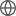Old Web
English
We present the discovery of PSO J083.8371+11.8482, a weak emission line quasar with extreme star formation rate at $z=6.3401$. This quasar was selected from Pan-STARRS1, UHS, and unWISE photometric data. Gemini/GNIRS spectroscopy follow-up indicates a MgII-based black hole mass of $M_\mathrm{BH}=\left(2.0^{+0.7}_{-0.4}\right)\times10^9~M_\odot$ and an Eddington ratio of $L_\mathrm{bol}/L_\mathrm{Edd}=0.5^{+0.1}_{-0.2}$, in line with actively accreting supermassive black hole (SMBH) at $z\gtrsim6$. HST imaging sets strong constraint on lens-boosting, showing no relevant effect on the apparent emission. The quasar is also observed as a pure point-source with no additional emission component. The broad line region (BLR) emission is intrinsically weak and not likely caused by an intervening absorber. We found rest-frame equivalent widths of EW(Ly$\alpha$+NV) $=5.7\pm0.7$ Angstrom, EW(CIV) $\leq5.8$ Angstrom (3-sigma upper limit), and EW(MgII) $=8.7\pm0.7$ Angstrom. A small proximity zone size ($R_\mathrm{p}=1.2\pm0.4$ pMpc) indicates a lifetime of only $t_\mathrm{Q}=10^{3.4\pm0.7}$ years from the last quasar phase ignition. ALMA shows extended [CII] emission with a mild velocity gradient. The inferred far-infrared luminosity ($L_\mathrm{FIR}=(1.2\pm0.1)\times10^{13}\,L_\odot$) is one of the highest among all known quasar hosts at $z\gtrsim6$. Dust and [CII] emissions put a constraint on the star formation rate of SFR $=900-4900~M_\odot\,\mathrm{yr^{-1}}$, similar to that of hyper-luminous infrared galaxy. Considering the observed quasar lifetime and BLR formation timescale, the weak-line profile in the quasar spectrum is most likely caused by a BLR which is not yet fully formed rather than continuum boosting by gravitational lensing or a soft continuum due to super-Eddington accretion.